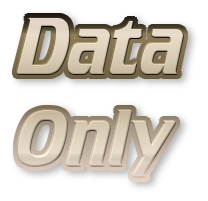# Unsupervised LearningUnsupervised learning is the aspect of machine learning that is concerned with approaches for learning from data where the outcomes are not available. The main goal of many unsupervised methods is to improve the interpretability of the data.

## Clustering

The goal of clustering is to assign each observation in a data set to a group based on the observed values associated with each observation. Different clustering approaches rely on different target functions and therefore lead to different cluster assignments. The following clustering algorithms are frequently used:

• $$k$$-means: assigns samples to $$k$$ clusters such that the distance to the mean value of the cluster is minimized.
• $$k$$-medoids: a clustering algorithm that is related to $$k$$-means that is more robust by relying on medoids rather than means.
• Spectral clustering: a clustering algorithm that represents samples as a graph.

## Dimensionality reduction

Unsupservised dimensionality reduction techniques aim at reducing the number of features while retaining as much information as possible. The following unsupervised dimensionality reduction methods are frequently used:

## Posts on unsupervised learning

The following posts discuss the use of unsupervised learning in R.

### Dimensionality Reduction for Visualization and Prediction

0

Dimensionality reduction is primarily used for exploring data and for reducing the feature space in machine learning applications. In this post, I investigate techniques such as PCA to obtain insights from a whiskey data set and show how PCA can be used to improve supervised approaches. Finally, I introduce the notion of the whiskey twilight zone.